# Introduction

Up to this point, we have used Markov chains to create a trail of agent locations distributed according to predefined steady state probability distribution. Now we want to use this tool to solve other problems.

## Using Markov chains to minimize a function

Imagine a function $$f(x)$$ defined on an interval $$(x_\text{min},x_\text{max})$$.
We want to find the minimum value of $$f$$.

f <- function(x){ x^2 }

Define a reasonably large number of states:

N <- 100

Define an interval:

xmin <- -3
xmax <-  3

Now create the $$x$$-values. These are the states the agent will visit.

xvals <- seq(xmin, xmax,len=N)

Take a peek at the plot of the values of the function on the sequence we just defined. The minimum is easy to see. We want to find it using a Markov chain!

plot(xvals, f(xvals), cex=.3)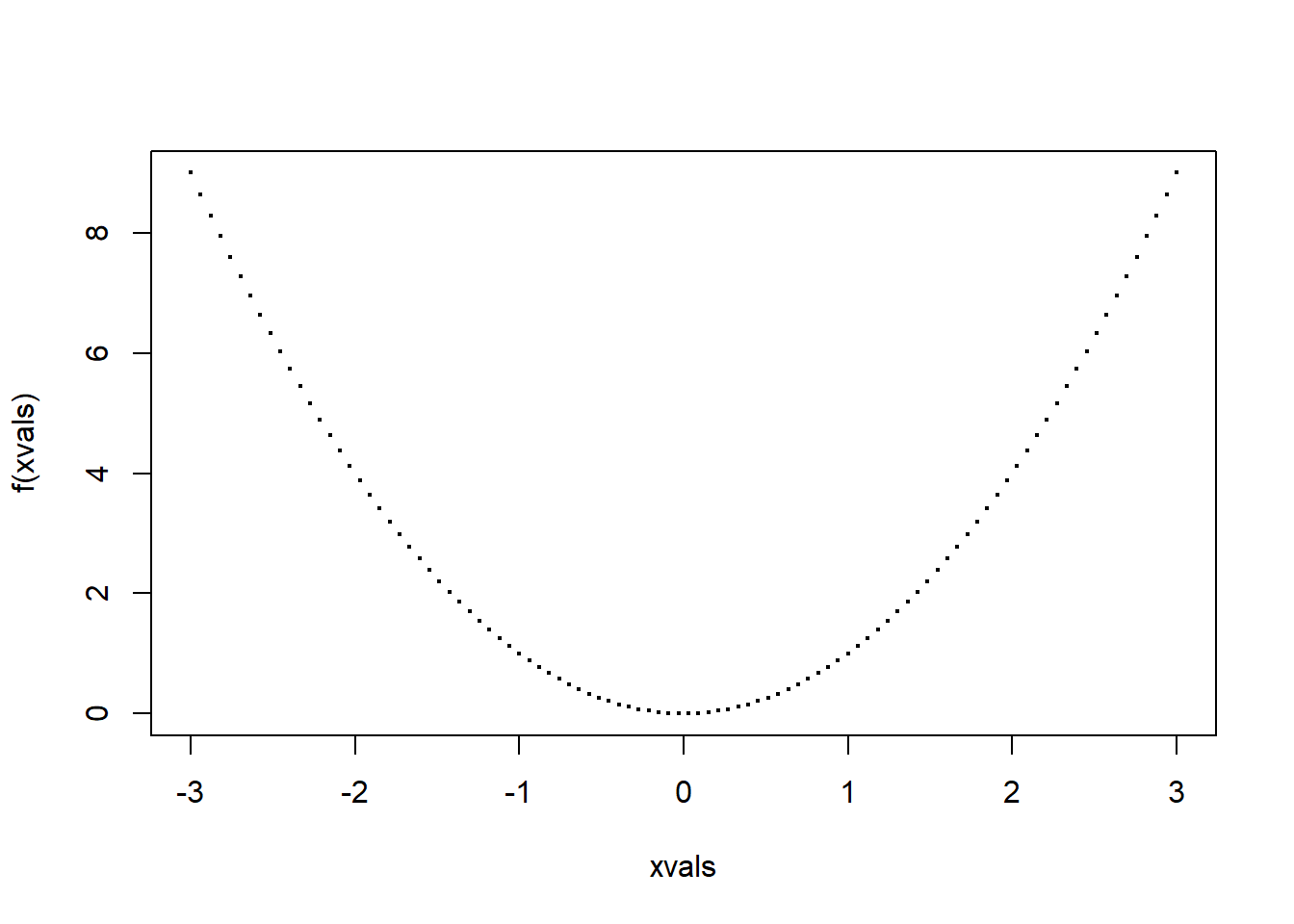Here’s the key step: From $$f(x)$$, we can create a probability distribution on the xvals, via $P(x) = e^{-f(x)/\sigma^2}$ where $$\sigma^2$$ represents the “variance”. In other words, $$1/sigma^2$$ is our precision.

The important thing here is that $$P$$ is maximimal where $$f$$ is minimal.

Let’s see what this means in practice. Create the probability function from $$f(x)$$. Note that the probability function depends on the choice of $$\sigma^2$$.

prob <- function(x,sig2) {
exp(-f(x)/sig2)
}

Let’s plot it for a few values of $$\sigma^2$$

sig2 <- 1
plot(xvals, prob(xvals, sig2), cex=.3)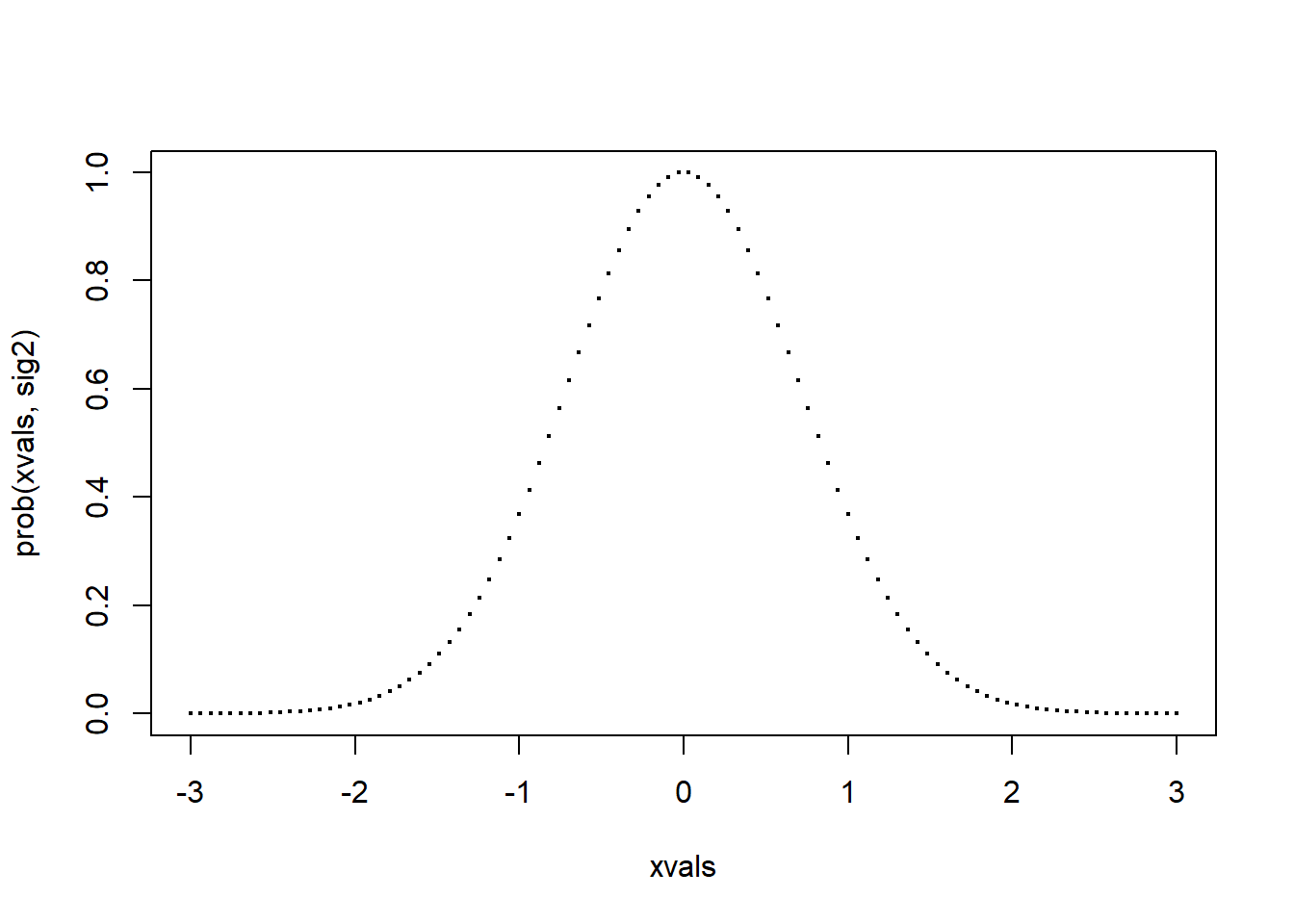Now with a smaller, or equivalently more precise, choice of $$\sigma^2$$.

sig2 <- 0.1
plot(xvals, prob(xvals, sig2), cex=.3)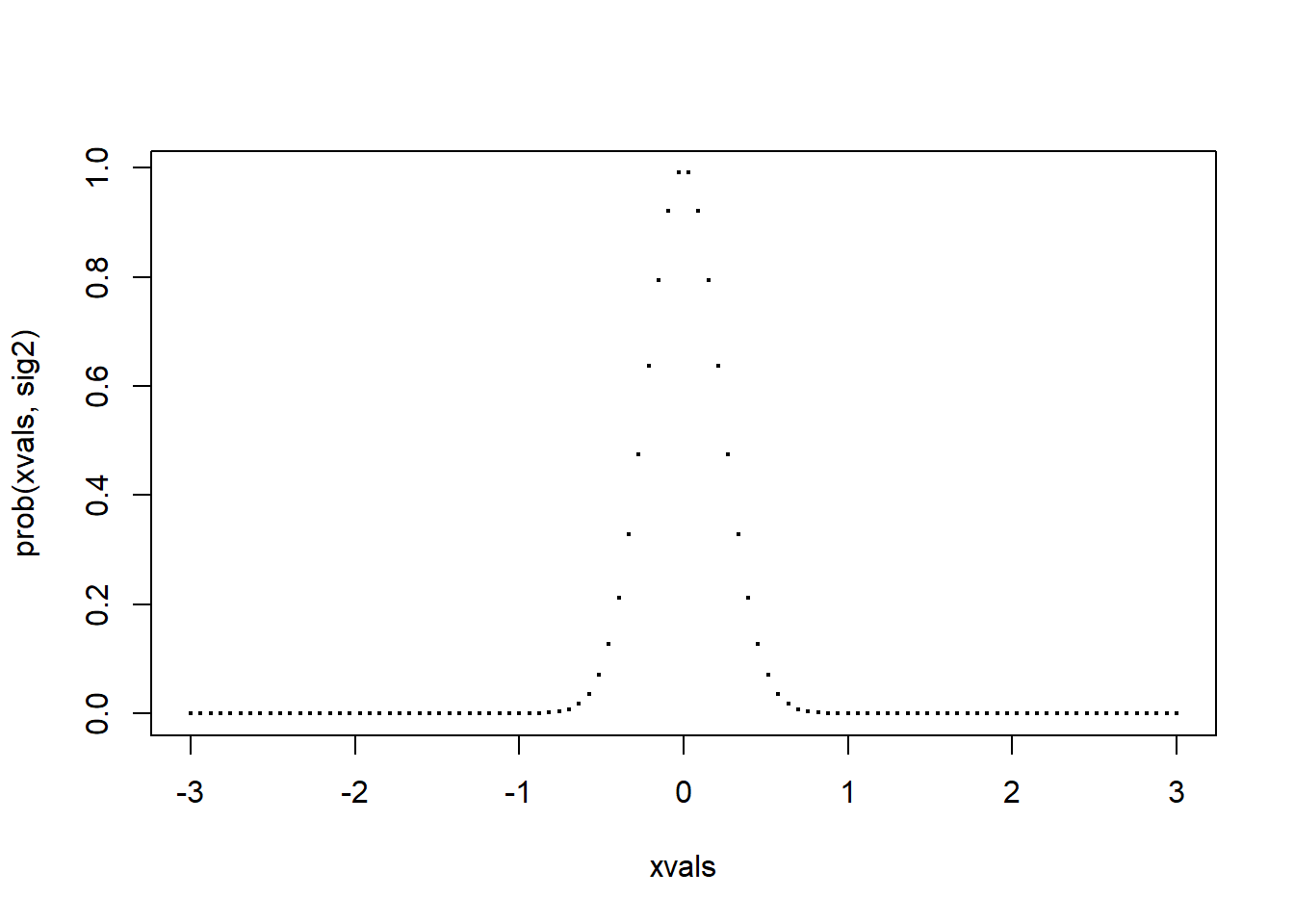Notice how these plots look like normal distributions. Also notice how much “tighter” the second plot is around its maximum value.

## Implement a Markov chain sampler.

We will start by creating a doMove function which depends on both the current state and the value of $$\sigma^2$$.

This function will work much like our move function from the last class. The algorithm will propose a transition to a new state, and then use a probabilistic approach that might or might not move to the proposed state.

Let $$P_1$$ represent the current probability and $$P_2$$ the proposed probability: \begin{aligned} P_1 &= e^{-\frac{f(x_1)}{\sigma^2}} \\ P_2 &= e^{-\frac{f(x_2)}{\sigma^2}} \end{aligned}

Notice how simple the ratio $$P_1/P_2$$ becomes.

As before, we will have the rule that if $$P_2 \ge P_1$$, then automatically make the transition. If $$P_2 < P_1$$, the make the transition with probability $\rho = \frac{P_2}{P_1} = e^{-\frac{f(x_2)-f(x_1)}{\sigma^2}}.$

Notice that we can compute this key ratio without computing the probabilities directly. We only need the difference in function values.

These two steps can be combined into a single statement.

• Regardless of the values of $$P_1$$ and $$P_2$$, define $$\rho$$ as above. Note that if $$P_2 > P_1$$, then $$\rho > 1$$.
• Generate a uniformly distributed random number $$r \in [0,1]$$. If $$r < \rho$$, then make the transition!

## Implementation

Here’s an implementation of this strategy. In this case, we will think of the state as one of the $$N$$ equally spaced values between (xmin, xmax).

First create a function that defines a proposal transition.

proposal <- function(currState){
# choose a proposal transition at random
delta <- sample(c(-1,1),1)/N
propState <- currState + delta
# avoid going off the end of the interval
if(propState <= xmin)
propState <- xmin
if(propState >= xmax)
propState <- xmax
propState
}

Now we can build a move. If you set things up right, this part almost always looks like this:

doMove <- function(currState,sig2){
# propose a transtion
propState<-proposal(currState)
# get function value difference
dFunc <- f(propState) - f(currState)
# calculate rho and make a move based on result
rho <- exp(-dFunc/sig2)
if(runif(1,0,1) < rho){
# return the proposed state (this will be the new state)
return(propState)
}
# return the old state (no transition)
return(currState)
}

## Try it out!

Set up: Define the number of steps (varies with the application), a vector to keep the states, an initial state, and a value of ^2\$.

M <- 100000
vals <- rep(0,M)
currState <- runif(1, xmin, xmax)
sig2 <- 1.0

Now the main loop.

for(m in 1:M){
vals[m] <- currState
currState <- doMove(currState, sig2)
}

Take a peek at the histogram of these values. It should look like a normal distribution with mean $$0$$ and variance $$\sigma^2.$$ The larger $$M$$ is, the more normal this should look.

hist(vals,breaks=100,xlim=c(xmin,xmax))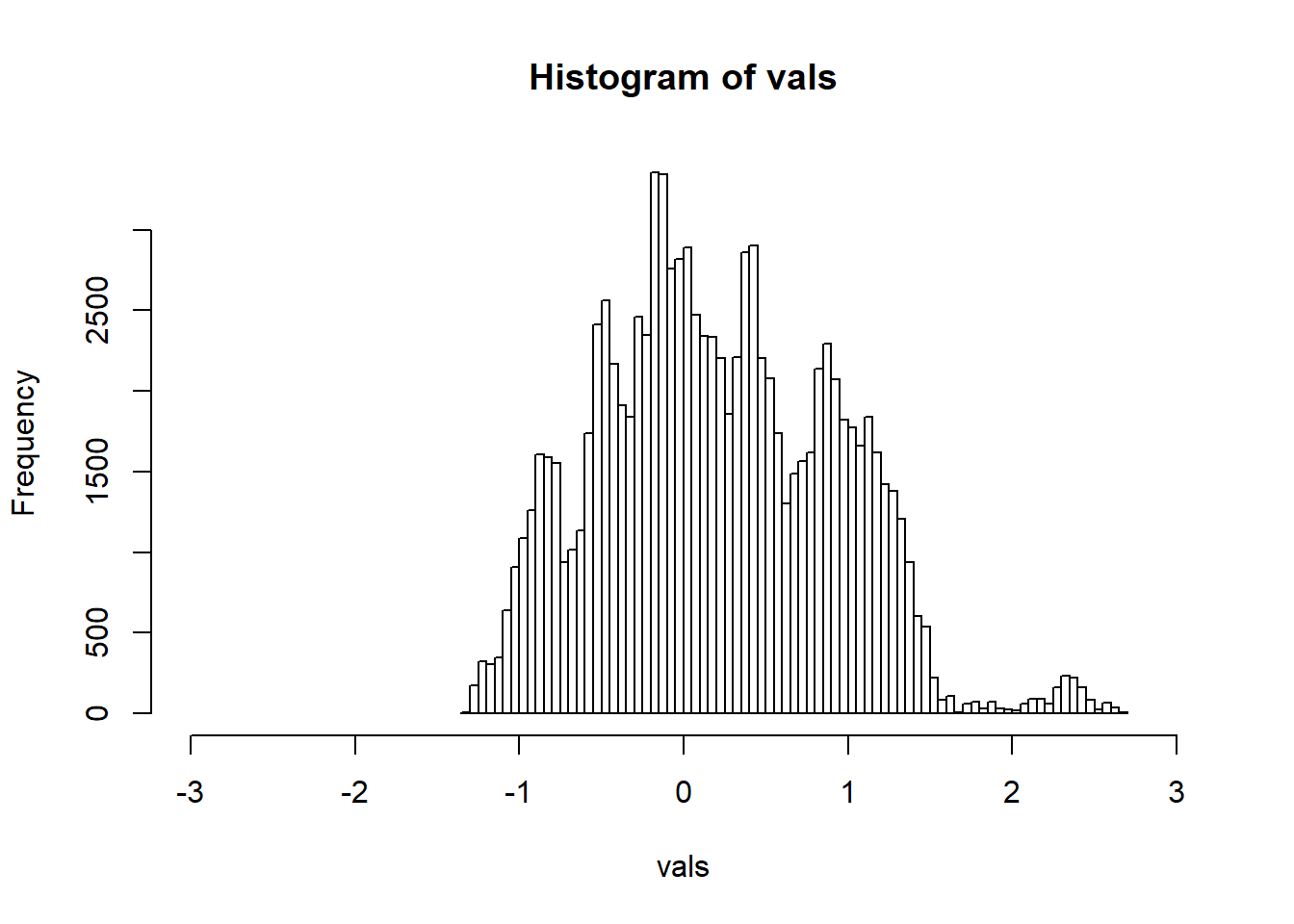A more revealing plot is the “trace” of the Markov chain states.

plot(vals, cex=.1)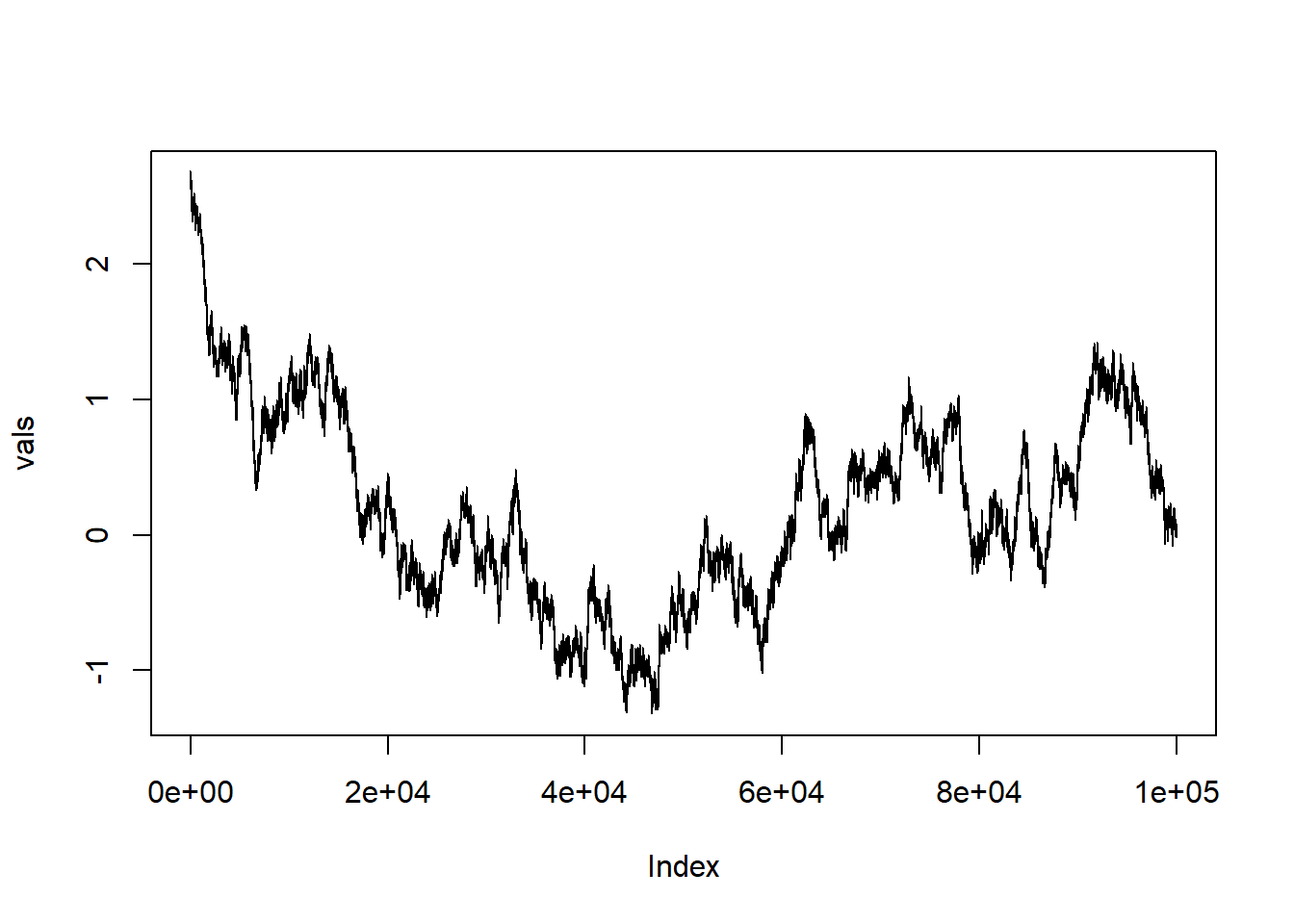The trace allows us to see how values of $$x$$ move around. Overall they represent a sample from the normal distribution with the fixed value of $$\sigma^2$$. The histogram peaks at the minimum value of $$f(x)$$, but there is a better way to identify this critical value.

# Simulated Annealing

Here is a clever idea: as the Markov chain moves along, slowly decrease $$\sigma^2$$, which means the probability distribution gets more tightly clustered around its maximum values. This is a way of “trapping” $$x$$ near the maximum value.

This process is called simulated annealing. The key difference is that $$\sigma^2$$ is slowly decreased as the chain moves along.

Here is another version of the previous code. Note the only difference is that now $$\sigma^2$$ slowly decreases.

M <- 100000
vals <- rep(0,M)
sig2 <- 1
decFac <- .9999
currState <- runif(1, xmin, xmax)
for(m in 1:M){
vals[m] <- currState
currState <- doMove(currState,sig2)
# slowly decrease sig2
sig2 <- sig2*decFac
}

Note: The method by which $$\sigma^2$$ decreases is art, not a science! Even in this simple case, you have to play around with both the initial value of $$\sigma^2$$ and the factor by which it decreases each step.

The histogram:

hist(vals,breaks=100,xlim=c(xmin,xmax))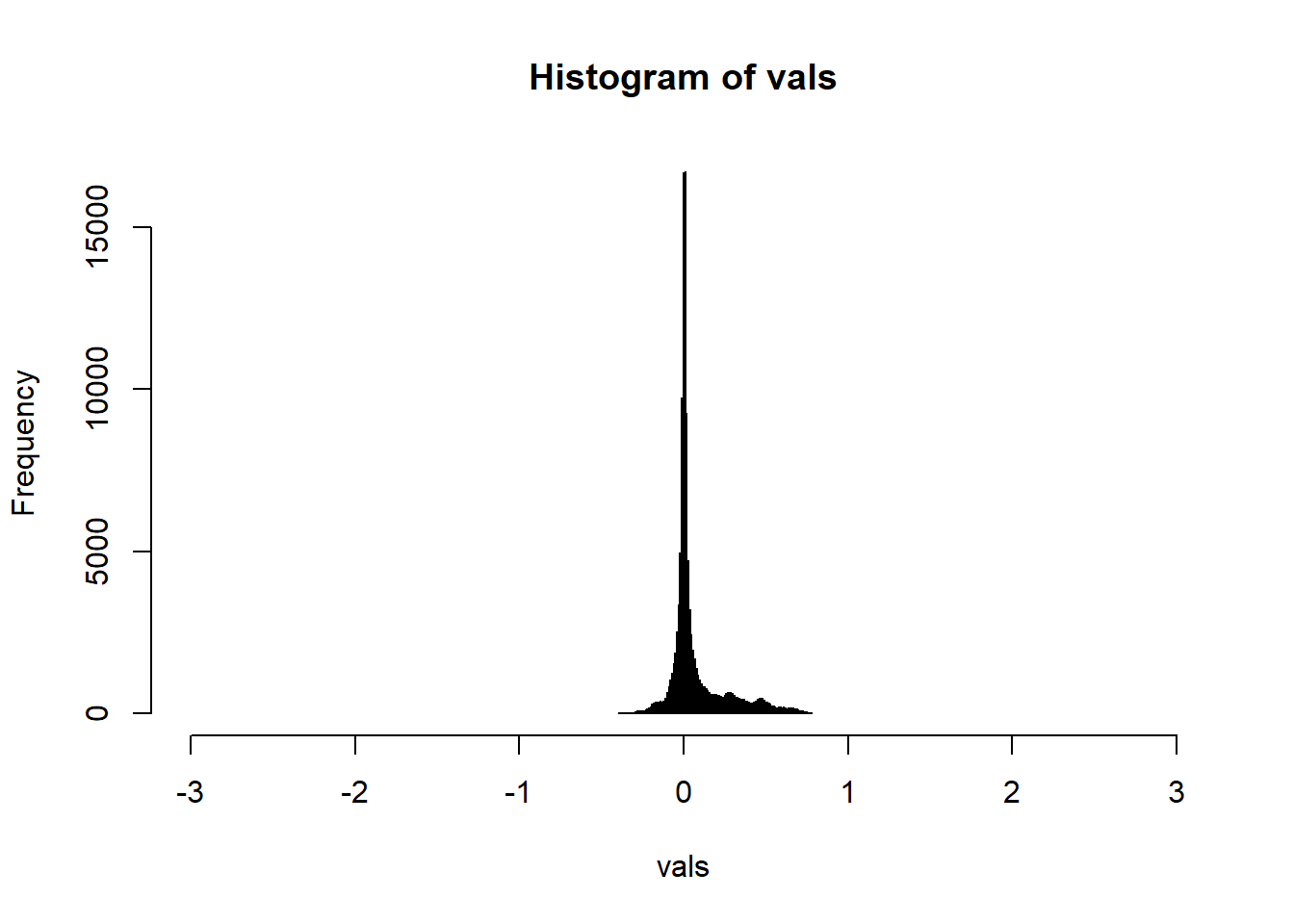The trace…this is most interesting:

plot(vals,cex=.1,type="l")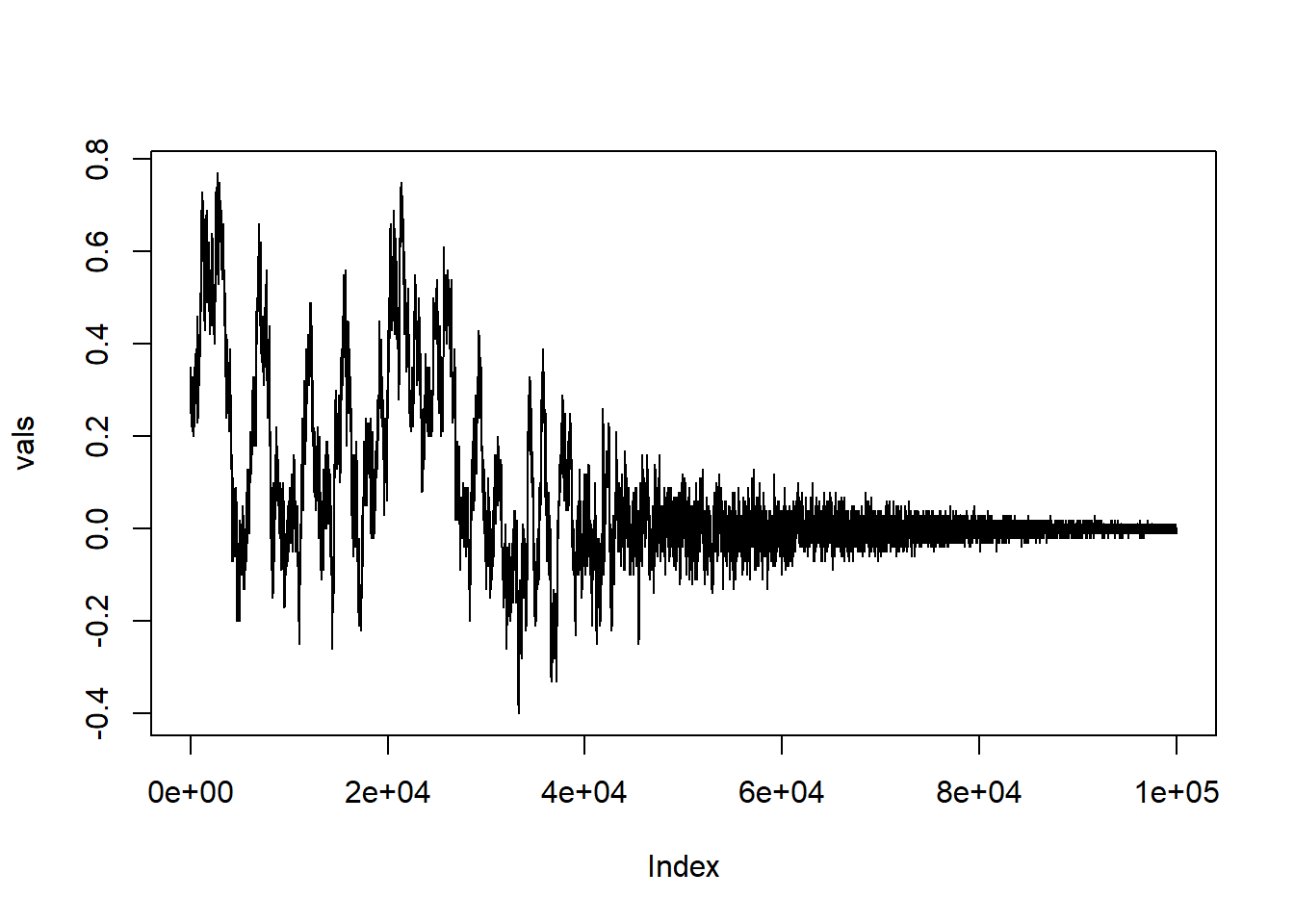Notice now that the trace of the Markov chains funnels down to the value $$x=0$$, which the minimum value of $$f(x) = x^2$$ on the interval $$(-3,3)$$.

You might think this is a hard way to find a the minimum of $$x^2$$. You’re right, but the idea has wonderful applications in more complicated minimization problems.

The key components are:

• A well defined state space for the state variable $$x$$.
• A natural proposal transition on the state space
• A function $$f(x)$$ on the state space that we want to minimize.

Implementation of simulated annealing can usually be broken into three steps.

• Create a proposal function. This very much depends on the problem under consideration.
• Create a doMove function. This often is very similar across applications
• Set up an initial value of $$\sigma^2$$ and a method to slowly decrease $$\sigma^2$$ as the main loop progresses.

With any luck, and some fiddling around, you can usually design this process so that minimum value of $$f(x)$$ is identified.

## Exercise: Repeat with a different function

This process can work for any function. We find a minimum of $$f(x)$$ by implementing the Markov chain agent model and then slowly decrease the value $$\sigma^2$$ and hope we lock onto the correct value.

Try it with the following function on the interval $$(-3,3)$$.

f <- function(x) {
(x-2)*(x-1)*x*(x-2.4)
}

Run your Markov chain several times and compare the output.

If you finish this before the end of class, try some other functions. Can you consistently find the minimum values?